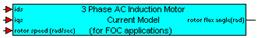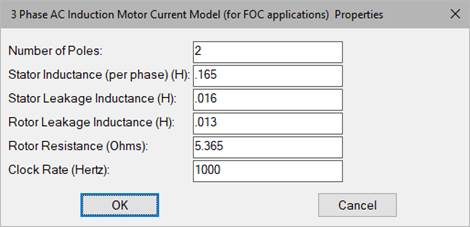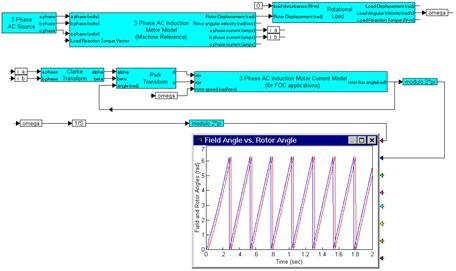### AC Induction Motor Current Model (FOC)Category: Controllers > Field Oriented Control

The AC Induction Motor Current Model (FOC) block is used for field-oriented control applications.

The AC Induction Motor Current Model (FOC) block uses sensed stator currents of an AC induction motor model (converted to DQ frame currents) and speed measurements to estimate the angular position of the stator magnetic field (direct axis) based on known motor parameters.Number of Poles: Requires the 3-phase AC induction motor number of pole pairs.

Stator Inductance (per phase): Requires the 3-phase AC induction motor stator per phase inductance in henries.

Stator Leakage Inductance: Requires the 3-phase AC induction motor specified stator leakage inductance in henries.

Rotor Leakage Inductance: Requires the specified 3-phase AC induction motor rotor leakage inductance in henries.

Rotor Resistance: Requires the 3-phase AC induction motor rotor winding resistance in ohms.

#### Example

Diagram name: ACIM FOC Model

Location: Examples > eMotor > AC Induction

The following simulation demonstrates how the field angle varies over time with respect to the rotor angle while operating the motor open loop at steady state velocity.